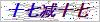Product center# MACK 截止阀/止回阀技术参数

MACK 截止阀/止回阀技术参数

MACK 截止阀/止回阀技术参数℃，（400℉）。

Fig. No.   Equiv. Cont. Check Valve

1132 = 3942

1138 = 3948

1532 = 3042

1538 = 3048

1632 = 3442

1651 = 3451

A2 1661 = 3461

A2 1672 = 3482

Fig. No.   Equiv. Cont. Check Valve

2132 = 4942

2138 = 4948

2532 = 4042

2538 = 4048

2632 = 4442

2651 = 4451

2661 = 4461

2672 = 4482

### 留言框

• #### 验证码：请输入计算结果（填写阿拉伯数字），如：三加四=7

QQ在线客服

• 021-37601287